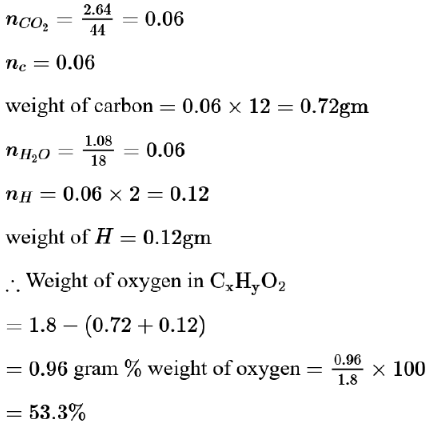# Complete combustion of 1.80 gQuestion:

Complete combustion of $1.80 \mathrm{~g}$ of an oxygen containing compound $\left(\mathrm{C}_{\mathrm{x}} \mathrm{H}_{\mathrm{y}} \mathrm{O}_{\mathrm{z}}\right)$ gave $2.64 \mathrm{~g}$ of $\mathrm{CO}_{2}$ and $1.08 \mathrm{~g}$ of $\mathrm{H}_{2} \mathrm{O}$. The percentage of oxygen in the organic compound is:

1. $63.53$

2. $53.33$

3. $51.63$

4. $50.33$

Correct Option: , 2

Solution: